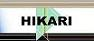| Home | Journals | Books | Paper submission | About us and our mission | News | Contact us |Site Search:

## Online Edition, Vol. 3, 2009, no. 33-36

Wanjuan Du, Zhongping Li, Li Xie
Neumann problem for coupled diffusion systems with localized nonlinear reactions
Int. Journal of Math. Analysis, Vol. 3, 2009, no. 33-36, 1599-1606.

Peng Lin, Gao Mingzhe
A note on Hardy-Hilbert type integral inequality
Int. Journal of Math. Analysis, Vol. 3, 2009, no. 33-36, 1607-1616.

H. A. Ghany
q-Derivative of basic hypergeometric series with respect to parameters
Int. Journal of Math. Analysis, Vol. 3, 2009, no. 33-36, 1617-1632.

S. Latha, O. Karthiyayini
Certain class of analytic and univalent functions involving the Ruscheweyh derivative operator
Int. Journal of Math. Analysis, Vol. 3, 2009, no. 33-36, 1633-1644.

M. A. Latif, M. Alomari
On Hadmard-type inequalities for h-convex functions on the co-ordinates
Int. Journal of Math. Analysis, Vol. 3, 2009, no. 33-36, 1645-1656.

Xiaolin Deng, Yafang Zhang, Peibiao Zhao
Portfolio optimization based on spectral risk measures
Int. Journal of Math. Analysis, Vol. 3, 2009, no. 33-36, 1657-1668.

S. Lal, H. P. Singh
Double matrix summability of double Fourier series
Int. Journal of Math. Analysis, Vol. 3, 2009, no. 33-36, 1669-1681.

Nguyen Buong, Nguyen Thi Thuy Hoa
Regularization for nonlinear complementarity problems
Int. Journal of Math. Analysis, Vol. 3, 2009, no. 33-36, 1683-1691.

Nguyen Buong, Nguyen Dinh Dung
Regularization for a common solution of a system of nonlinear ill-posed equations
Int. Journal of Math. Analysis, Vol. 3, 2009, no. 33-36, 1693-1699.

B. Xh. Fejzullahu, R. Xh. Zejnullahu
Lebesgue constants for Fourier expansions associated with weight function (1-x)^\alpha (1+x)^\beta+M\delta_{-1}+N\delta_{1}
Int. Journal of Math. Analysis, Vol. 3, 2009, no. 33-36, 1701-1709.

Vu Van Khuong
Qualitative properties for a fourth-order rational difference equation (I)
Int. Journal of Math. Analysis, Vol. 3, 2009, no. 33-36, 1711-1722.

Vu Van Khuong
Qualitative properties for a fourth-order rational difference equation (III)
Int. Journal of Math. Analysis, Vol. 3, 2009, no. 33-36, 1723-1733.

F. O. Nyamwala
Norms of symmetrised two-sided multiplication operators
Int. Journal of Math. Analysis, Vol. 3, 2009, no. 33-36, 1735-1744.

S. Imnang, S. Suantai
Approximating common fixed points for a finite family of non-Lipschitzian mappings in Banach spaces
Int. Journal of Math. Analysis, Vol. 3, 2009, no. 33-36, 1745-1759.

K. Chandrasekhara Rao, S. Tamilselvan, K. Vairamanickam
Some vector sequence spaces-II
Int. Journal of Math. Analysis, Vol. 3, 2009, no. 33-36, 1761-1768.

Numerical solution of Fredholm integro-differential equation by Adomian's decomposition method
Int. Journal of Math. Analysis, Vol. 3, 2009, no. 33-36, 1769-1773.

M. Pasic, A. Raguz
Singular behaviour of bounded radially symmetric solutions of p-Laplace nonlinear equation
Int. Journal of Math. Analysis, Vol. 3, 2009, no. 33-36, 1775-1788.

I. Braja
Characterizations of regular gamma semi-groups using quazi-ideals
Int. Journal of Math. Analysis, Vol. 3, 2009, no. 33-36, 1789-1794.

M. Hazarika, B. Kalita
A generalized definition of joint hyponormality
Int. Journal of Math. Analysis, Vol. 3, 2009, no. 33-36, 1795-1802.